### Puzzle # 6 - Find a number that fits these rules.

Find a six-digit number in which:

1) the first digit is three less than the second and five less than the third,

2) the third digit is two less than the fourth,

3) the fifth digit is six less than the fourth,

4) the last digit is double the fifth and,

5) the sum of all the digits is 32.

___ ___ ___ ___ ___ ___
1st- 2nd- 3rd- 4th- 5th- 6th

[HINT: Each digit can only be 0, 1, 2, 3, 4, 5, 6, 7, 8 or 9. Cut out ten squares of paper and write each of these digits on one square. Play around with rearranging the numbers in order to see where you might put each digit. Only 6 of these 10 numbers are used and they are only used once, no number is repeated.]

### Puzzle #5

Puzzle #5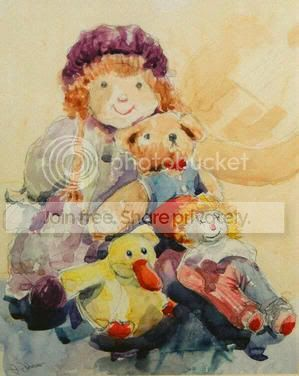[Image from Julia Johnson: http://www.westwycombepainters.com/Julia-Johnson]

Rebecca wants to get rid of most of her dolls.
She gave one-fourth of her collection, plus 10, to Nancy.
Then she gave one-fifth of what was left, plus 10, to Susy, and half of what was left, plus 10, to Cheryl.
She kept the last 5.

How many dolls did Rebecca start out with?

)

### Puzzle #4

Puzzle #4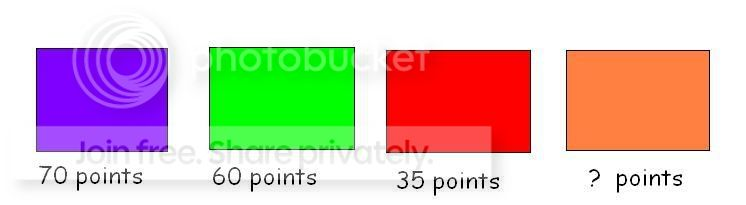If purple is worth 70 points, green is worth 60 points and red is worth 35 points, you can find out what any colour word is worth in points using the same scoring system.

How many points would orange be worth?

[Hint: One way might be to think about how much each letter in the colour word might be worth.  Then get a theory that works for one of the words and then check to see if it works for the other two colour words you know.]

This one's tricky and there is more than one answer.  As long as you can find a rule that works, you're right!

### Puzzle # 3

Puzzle # 3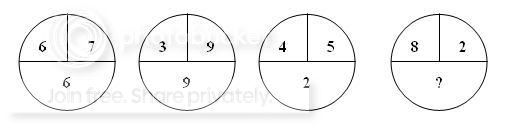In the puzzle above, there are three circles. The two parts of the top of the circle go together somehow to make the bottom of the circle.

Can you figure out the number pattern and tell me what the number in the bottom of the third circle should be?

[Hint: Try the different operations: addition, subtraction, multiplication and division, to see if you can get the two top numbers to equal the bottom number in the first circle. Then test your theory on the second circle!]

If you think you have the answer to a puzzle, then email me your answer, telling me which puzzle number it is (e.g. Puzzle #3).

Feel free to swap ideas with each other in the forum by posting a reply to this post! Have fun!

### Puzzle #2

Puzzle #2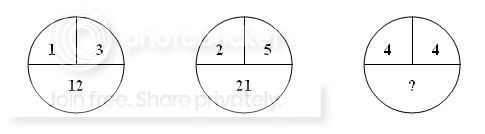In the puzzle above, there are three circles.  The two parts of the top of the circle go together somehow to make the bottom of the circle.

Can you figure out the number pattern and tell me what the number in the bottom of the third circle should be?

[Hint: Try the different operations: addition, subtraction, multiplication and division, to see if you can get the two top numbers to equal the bottom number in the first circle.  Then test your theory on the second circle!]

If you think you have the answer to a puzzle, then email me your answer, telling me which puzzle number it is (e.g. Puzzle #2).

Feel free to swap ideas with each other in the forum by posting a reply to this post! Have fun!

### Welcome to the Mathematics Community

This community is primarily for:

KS3 and KS4/GCSE ~~~~ 11-16 year old students who want help with revision or to push their thinking with puzzles.

Teachers
~~~~ Who may want puzzle ideas for lesson starters or to direct students towards this site for out-of-school maths help.

You can use this community for:

Homework help ~~~~ Just post a message and anyone is allowed to help answer your question.

Maths puzzles for fun ~~~~
I suggest you don't post your answer to the puzzle in the comments so others have the chance to try it for themselves.  And if you want to try a puzzle on your own without any help first, then I suggest you don't look at the comments before you attempt the puzzle^_^

If you think you have the answer to a puzzle, then email me your answer, telling me which puzzle number it is (e.g. Puzzle #1).

Feel free to comment on a puzzle post. Tell me whether you enjoyed it, thought it was too easy or too hard or would like more like it!

### Puzzle # 1

Puzzle #1

Matchstick problem:

This is a glass with a maraschino cherry in it. (Yum, my favourite for on top of sundaes!) By only moving two matchsticks, can you turn the glass upside-down leaving the cherry outside of the glass?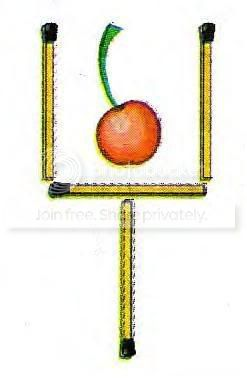[Suggestion: Get some pens/pencils and make the shape so you can play with moving the matchsticks around.]

If you think you have the answer to a puzzle, then email me your answer, telling me which puzzle number it is (e.g. Puzzle #1).accomplished